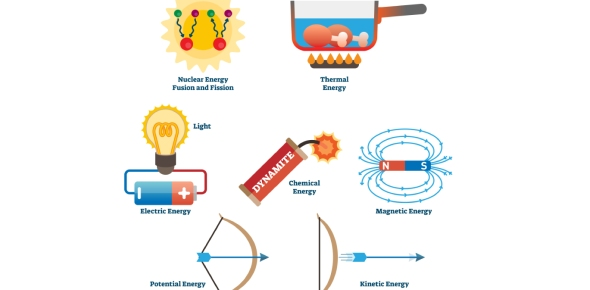# Block 1 Energy Resources And Forms Of Energy

20 Questions | Total Attempts: 35Settings• 1.
The energy of a moving object is
• A.

Kinetic energy

• B.

Potential energy

• C.

Chemical energy

• D.

Solar energy

• 2.
. The law of conservation of energy states that
• A.

Energy cannot be converted from one form to another

• B.

Energy cannot be created or destroyed

• C.

Energy resources must be used efficiently

• D.

Energy is constantly being lost to friction

• 3.
Which form of energy does a plant store when light is transformed during photosynthesis?
• A.

Solar energy

• B.

Nuclear energy

• C.

Mechanical energy

• D.

Chemical energy

• 4.
According to the law of conservation of energy, what happens to the total amount of energy in the universe?
• A.

Increases

• B.

Decreases

• C.

Remains constant

• D.

Changes constantly

• 5.
Organic material like plants.
• A.

Nuclear power

• B.

Biomass

• C.

Solar energy

• D.

Geothermal energy

• 6.
Heat produced in earth’s core from radioactive decay.
• A.

Biomass

• B.

Nuclear

• C.

Geothermal

• D.

Coal

• 7.
Moving air caused by uneven heating of Earth’s surface
• A.

Hydropower

• B.

Solar energy

• C.

Wind energy

• D.

Geothermal energy

• 8.
Fossil fuel, colorless, odorless gas
• A.

Petroleum

• B.

Natural gas

• C.

Coal

• D.

Nuclear power

• 9.
Solid fossil fuel
• A.

Petroleum

• B.

Coal

• C.

Natural gas

• D.

Solar

• 10.
Biomass is a _____________________ energy resource
• A.

Nonrenewable

• B.

Renewable

• 11.
Nuclear power is a ____________________________ energy resource
• A.

Non renewable

• B.

Renewable

• 12.
Geothermal power is a _______________________ energy resource.
• A.

Non-renewable

• B.

Renewable

• 13.
Form of energy that in which vibrations travel as waves
• A.

Heat

• B.

Sound

• C.

Chemical

• 14.
Movement of particles in matter, increased movement or particles, more heat is released
• A.

Chemical

• B.

Solar

• C.

Thermal

• D.

Nuclear

• 15.
Forces between charged particles
• A.

Nuclear energy

• B.

• C.

Electrical energy

• D.

Thermal energy

• 16.
Energy is converted from one form to another
• A.

Energy efficiency

• B.

Energy conversion

• C.

Energy resource

• 17.
There is a direction connection between work and energy.
• A.

True

• B.

False

• 18.
Gas is a what form of energy
• A.

Mechanical

• B.

Chemical

• C.

Thermal

• D.

Mechanical

• 19.
When gas is burned in an engine it converts to what type of energy.
• A.

Chemical

• B.

Thermal or heat

• C.

Electrical

• D.

Solar

• 20.
20. Consider the energy conversion changes and choose the correct order of energy changes.  Sun shines on grass.  Cow eats grass.  Cow moos.
• A.

Sound, chemical, mechanical, thermal, solar

• B.

Nuclear, solar, chemical, thermal, mechanical

• C.Back to top